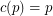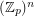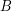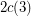Importance: High ✭✭✭
 Author(s): Jaeger, Francois Linial, Nathan Payan, Charles Tarsi, Michael
 Subject: Combinatorics » Matrices
Conjecture   For every prime, there is a constant(possibly) so that the union (as multisets) of anybases of the vector spacecontains an additive basis.
Definition: Letbe a finite dimensional vector space over the field. We call a multisetwith elements inan additive basis if for every, there is a subset ofwhich sums to.
It is worth noting that this conjecture would also imply that every-edge-connected graph has a nowhere-zero 3-flow, thus resolving The weak 3-flow conjecture.